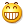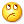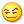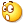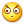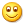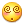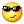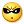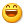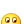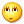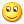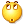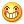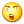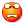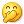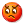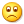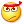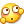<转载>20 个 Laravel Eloquent 必备的实用技巧
in LaravelPHP with 0 comment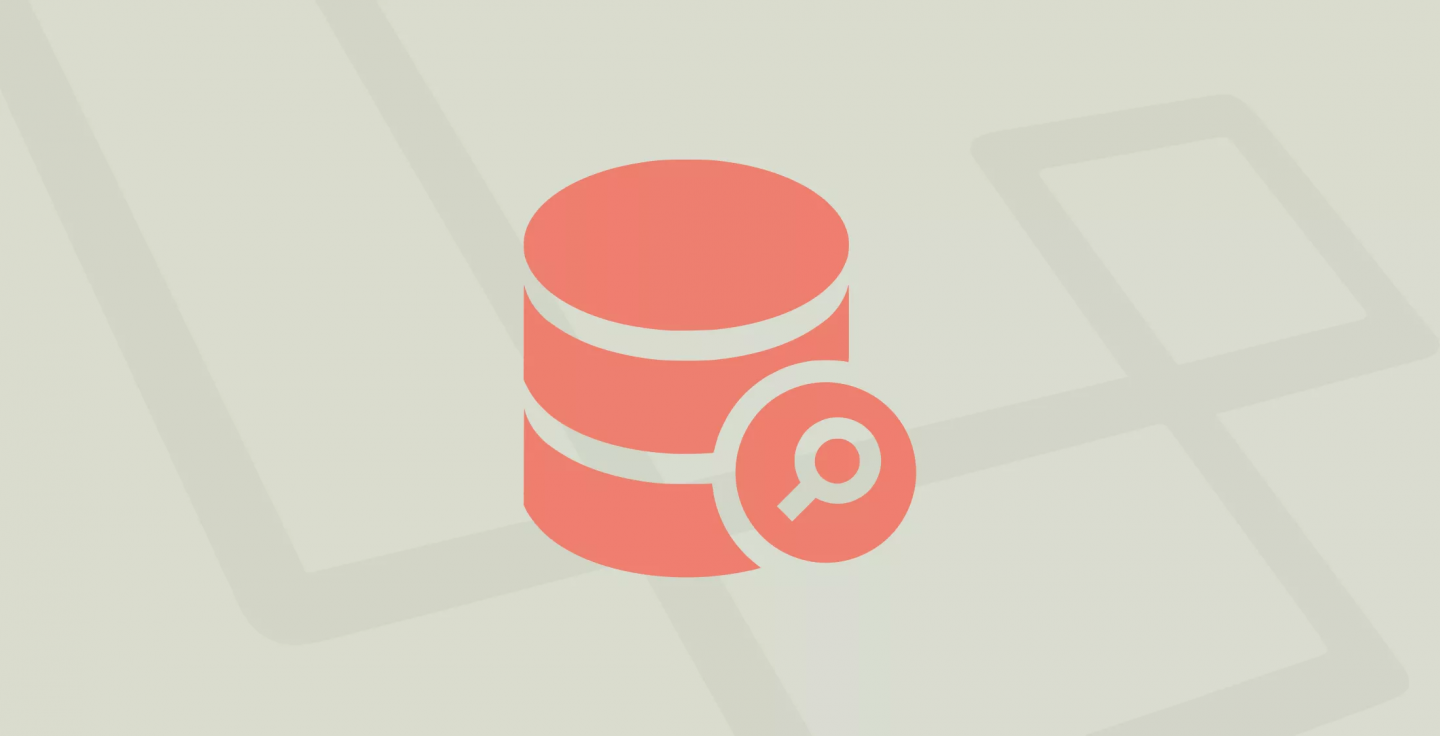Eloquent ORM 看起来是一个简单的机制，但是在底层，有很多半隐藏的函数和鲜为人知的方式来实现更多功能。在这篇文章中，我将演示几个小技巧。

### 递增和递减

``````\$article = Article::find(\$article_id);
\$article->save();``````

``````\$article = Article::find(\$article_id);

``````Article::find(\$article_id)->increment('read_count');
Product::find(\$produce_id)->decrement('stock'); // -1``````

### 先执行 X 方法，X 方法执行不成功则执行 Y 方法

Eloquent 有相当一部分函数可以把两个方法结合在一起使用，例如 『 请先执行 X 方法， X 方法执行不成功则执行 Y 方法 』。

``````\$user = User::find(\$id);
if (!\$user) { abort (404); }``````

``\$user = User::findOrFail(\$id);``

``````\$user = User::where('email', \$email)->first();
if (!\$user) {
User::create([
'email' => \$email
]);
}``````

``\$user = User::firstOrCreate(['email' => \$email]);``

### 模型的 boot () 方法

``````class User extends Model
{
public static function boot()
{
parent::boot();
static::updating(function(\$model)
{
// 写点日志啥的
// 覆盖一些属性，类似这样 \$model->something = transform(\$something);
});
}
}``````

``````public static function boot()
{
parent::boot();
self::creating(function (\$model) {
\$model->uuid = (string)Uuid::generate();
});
}``````

### 带条件与排序的关联关系

``````public function users() {
return \$this->hasMany('App\User');
}``````

``````public function approvedUsers() {
return \$this->hasMany('App\User')->where('approved', 1)->orderBy('email');
}``````

### 模型特性：时间、追加等

Eloquent 模型有些参数，使用类的属性形式。最常用是：

``````class User extends Model {
protected \$table = 'users';
protected \$fillable = ['email', 'password']; // 可以被批量赋值字段，如 User::create() 新增时，可使用字段
protected \$dates = ['created_at', 'deleted_at']; // 需要被Carbon维护的字段名
protected \$appends = ['field1', 'field2']; // json返回时，附加的字段
}``````

``````protected \$primaryKey = 'uuid'; // 更换主键
public \$incrementing = false; // 设置 不自增长
protected \$perPage = 25; // 定义分页每页显示数量（默认15）
const CREATED_AT = 'created_at';
const UPDATED_AT = 'updated_at'; //重写 时间字段名
public \$timestamps = false; // 设置不需要维护时间字段``````

### 通过 ID 查询多条记录

``\$user = User::find(1);``

``\$users = User::find([1,2,3]);``

### WhereX

``\$users = User::where('approved', 1)->get();``

``\$users = User::whereApproved(1)->get();``

``````User::whereDate('created_at', date('Y-m-d'));
User::whereDay('created_at', date('d'));
User::whereMonth('created_at', date('m'));
User::whereYear('created_at', date('Y'));``````

### 通过关系排序

``````public function latestPost()
{
return \$this->hasOne(\App\Post::class)->latest();
}``````

``\$users = Topic::with('latestPost')->get()->sortByDesc('latestPost.created_at');``

### Eloquent::when () -- 不再使用 if-else

``````if (request('filter_by') == 'likes') {
\$query->where('likes', '>', request('likes_amount', 0));
}
if (request('filter_by') == 'date') {
\$query->orderBy('created_at', request('ordering_rule', 'desc'));
}``````

``````\$query = Author::query();
\$query->when(request('filter_by') == 'likes', function (\$q) {
return \$q->where('likes', '>', request('likes_amount', 0));
});
\$query->when(request('filter_by') == 'date', function (\$q) {
return \$q->orderBy('created_at', request('ordering_rule', 'desc'));
});``````

``````\$query = User::query();
\$query->when(request('role', false), function (\$q, \$role) {
return \$q->where('role_id', \$role);
});
\$authors = \$query->get();``````

### 一对多返回默认模型对象

``{{ \$post->author->name }}``

``{{ \$post->author->name ?? '' }}``

``````public function author()
{
return \$this->belongsTo('App\Author')->withDefault();
}``````

``````public function author()
{
return \$this->belongsTo('App\Author')->withDefault([
'name' => 'Guest Author'
]);
}``````

### 通过赋值函数进行排序

``````function getFullNameAttribute()
{
return \$this->attributes['first_name'] . ' ' . \$this->attributes['last_name'];
}``````

``\$clients = Client::orderBy('full_name')->get(); //没有效果``

``\$clients = Client::get()->sortBy('full_name'); // 成功!``

### 全局作用域下的默认排序

``````protected static function boot()
{
parent::boot();

// 按照 name 正序排序
\$builder->orderBy('name', 'asc');
});
}``````

### 原生查询方法

``````// whereRaw
\$orders = DB::table('orders')
->whereRaw('price > IF(state = "TX", ?, 100)', )
->get();

// havingRaw
Product::groupBy('category_id')->havingRaw('COUNT(*) > 1')->get();

// orderByRaw
User::where('created_at', '>', '2016-01-01')
->orderByRaw('(updated_at - created_at) desc')
->get();``````

### 复制：复制一行的副本

``````\$task = Tasks::find(1);

### Chunk () 方法之大块数据

``````\$users = User::all();
foreach (\$users as \$user) {
// ...``````

``````User::chunk(100, function (\$users) {
foreach (\$users as \$user) {
// ...
}
});``````

### 创建模型时创建额外的东西

``php artisan make:model Company``

``php artisan make:model Company -mcr``
• -m 将创建一个迁移文件
• -c 将创建一个控制器
• -r 表示控制器应该是一个资源控制器

### 调用 save 方法的时候指定 updated_at

``````\$product = Product::find(\$id);
\$product->updated_at = '2019-01-01 10:00:00';
\$product->save(['timestamps' => false]);``````

### update () 的结果是什么？

``\$result = \$products->whereNull('category_id')->update(['category_id' => 2]);``

### 把括号转换成 Eloquent 查询

``... WHERE (gender = 'Male' and age >= 18) or (gender = 'Female' and age >= 65)``

``````\$q->where('gender', 'Male');
\$q->orWhere('age', '>=', 18);
\$q->where('gender', 'Female');
\$q->orWhere('age', '>=', 65);``````

``````\$q->where(function (\$query) {
\$query->where('gender', 'Male')
->where('age', '>=', 18);
})->orWhere(function(\$query) {
\$query->where('gender', 'Female')
->where('age', '>=', 65);
})``````

### 复数参数的 orWhere

``````\$q->where('a', 1);
\$q->orWhere('b', 2);
\$q->orWhere('c', 3);``````

``````\$q->where('a', 1);
\$q->orWhere(['b' => 2, 'c' => 3]);``````

Responses